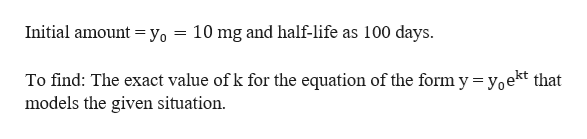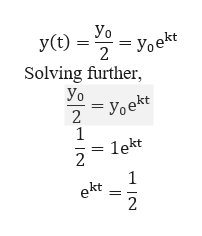# I1the population toA.Evaluatey= 2e )2. When will the population reach 3 million?B. Solve 2e.026.3. How large will the population be in 3 yr?C.Evaluate y= 2e023)4. How large will the population be in 4 months?D.Solve 2e.02 3CONCEPT PREVIEW Radioactive Decay Strontium-90 decays according to the expo-nential functionyyoe 0.0241where t is time in years. Match each question in Column I with the correct procedure inColumn Il to answer thequestion.III5. If the initial amount of Strontium-90 is 200 g,A.Solve 0.75yo yoe0.0241thow much will remain after 10 yr?6. If the initial amount of Strontium-90 is 200 g,how much will remain after 20 yr?B.Evaluate y = 200e .0241 (10)7. What is the half-life of Strontium-90?C. Solve yo yoe 0.0241r.8. How long will it take for any amount ofStrontium-90 to decay to 75% of its initialD.Evaluate y = 200e 0.0241 (20)amount?(Modeling)exponential or logarithmic models. See Examples 1-6.The exercises in this set are grouped according to discipline. They involvePhysical Sciences (Exercises 9-28)An initial amount of a radioactive substance yo is given, along with information aboutthe amount remaining after a given time t in appropriate units. For an equation of theform y = yo ekt that mode ls the situation, give the exact value of k in terms of naturallogarithms.After 6 hr, 10 g remain.10. Уo -30gs9. yo= 60 g; After 3 hr, 20 g remain.12. yo = 20 mg; The half-life is 200 days.11. yo = 10 mg; The half-life is 100 days.14. yo= 8.1 kg; After 4 yr, O.9 kg remains.2.4 lb; After 2 yr, 0.6 lb remains.13. yo15. Decay of Lead A sample of 500 g of radioactive lead-210 decays to polonium-210according to the functionSolve each problem.A(t) = 500e 0.032;where t is time in years. Find the amount of radioactive lead remaining after(c) 20 yr.(d) Find the half-life.(b) 8 yr,(а) 4 yr,ల

Question
19 views

check_circle

Step 1

Given that a radioactive element with the following:help_outlineImage Transcriptionclose10 mg and half-life as 100 days. Initial amount = yo To find: The exact value of k for the equation of the form y = yoekt that models the given situation fullscreen
Step 2

The half-life of a radioactive material is the time required to decay to one-half of the initial value of the material.help_outlineImage TranscriptioncloseУo Уoekt 2 Solving further Уo - Уоekt 2 1 lekt 2 1 ekt 2 fullscreen
Step 3

Now, given that the half life of the given element...help_outlineImage Transcriptionclose1 ek(100) 2 Taking log on both sides, In(ek(100)) In 100k In(e) n 100k n 1 In k = 100 fullscreen

### Want to see the full answer?

See Solution

#### Want to see this answer and more?

Solutions are written by subject experts who are available 24/7. Questions are typically answered within 1 hour.*

See Solution
*Response times may vary by subject and question.
Tagged in